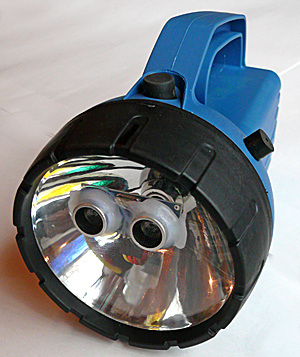i have already coding my arduino to sound the buzzer when ultrasonic sensor detect the object, but i cant solve the problem.. the ultrasonic sensor already detect object, but the buzzer not give the soud when the ultrasonic detect the object..... here is my code,

#include <Servo.h>

const int trigPin=9;
const int echoPin=10;
const int buzzer = 13;

long duration;
int distance;

Servo s1;

void setup() {
pinMode(buzzer, OUTPUT);
Serial.begin(9600);
pinMode(trigPin,OUTPUT);
pinMode(echoPin,INPUT);

s1.attach(11);
}

void loop()

{
for(int i=15;i<=60;i++){ // rotates the servo motor from 15 to 165 degrees
s1.write(i);
delay(10);
distance = calDist();

Serial.print(i); // Sends the current degree into the Serial Port
Serial.print(","); // Sends addition character right next to the previous value needed later in the Processing IDE for indexing
Serial.print(distance); // Sends the distance value into the Serial Port
Serial.print("."); // Sends addition character right next to the previous value needed later in the Processing IDE for indexing
}

for(int i=60;i>15;i--){
s1.write(i);
delay(10);
distance = calDist();
Serial.print(i);
Serial.print(",");
Serial.print(distance);
Serial.print(".");
}

}

int calDist()
{
digitalWrite(trigPin, LOW);
delayMicroseconds(2);
// Sets the trigPin on HIGH state for 10 micro seconds
digitalWrite(trigPin, HIGH);
delayMicroseconds(10);
digitalWrite(trigPin, LOW);
duration = pulseIn(echoPin, HIGH);
// Calculating the distance
distance= duration*0.034/2; // Range : 2cm to 400 cm
if (distance = 500){
tone(buzzer, 2500);
delay(500);
tone (buzzer, 2500);
}
else{
noTone (buzzer);
}
return distance;
}

please help meYou seem to have posted the code twice.

Please remember to use code tags when posting code.

`if (distance = 500){`Oops

sorry to write twice the code, i already update my code once... can you give me solution where i did write the wrong code for sound the buzzer?

thanks

Hi,

There is working code here that controls a buzzer from Ultrasonic..

https://arduinoinfo.mywikis.net/wiki/Project-HandBat